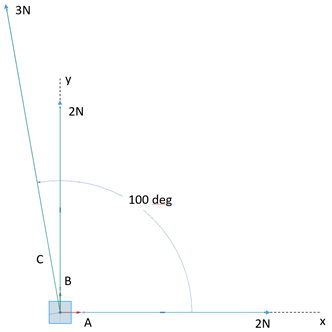## Vector Magnitudes

Calculate the resultant magnitude from the three coplanar forces below.Hint
$$R=\sqrt{{R_{x}}^{2}+{R_{y}}^{2}}$$$Hint 2 To find the total forces both in the x and y directions: $$R_{x}=\sum F_{xi}$$$
$$R_{y}=\sum F_{yi}$$$$$i=A,B,C$$$
To find the total forces both in the x and y directions.
$$R_{x}=\sum F_{xi}$$$$$R_{y}=\sum F_{yi}$$$
$$i=A,B,C$$$$$R_{x}=2N+3cos(100)N+0N=2N-0.52N=1.47N$$$
$$R_{y}=2N+3 sin⁡(100)N+0N=2N+2.95N=4.95N$$$Finally, to solve for the magnitude: $$R=\sqrt{{R_{x}}^{2}+{R_{y}}^{2}}=\sqrt{(1.47N)^{2}+(4.95N)^{2}}=\sqrt{26.67N^{2}}=5.16\:N$$$
5.16 N